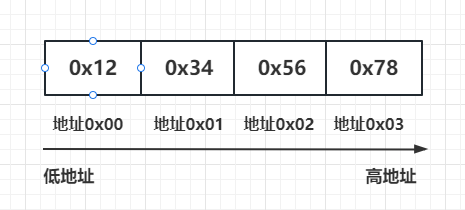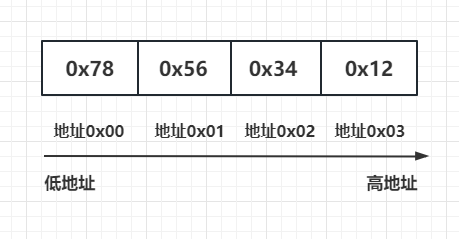# 恋恋风辰的官方博客

## 如何区分本机字节序

``#include <iostream>``using namespace std;``// 判断当前系统的字节序是大端序还是小端序``bool is_big_endian() {``    int num = 1;``    if (*(char*)&num == 1) {``        // 当前系统为小端序``        return false;``    } else {``        // 当前系统为大端序``        return true;``    }``}``int main() {``    int num = 0x12345678;``    char* p = (char*)&num;``    cout << "原始数据：" << hex << num << endl;``    if (is_big_endian()) {``        cout << "当前系统为大端序" << endl;``        cout << "字节序为：";``        for (int i = 0; i < sizeof(num); i++) {``            cout << hex << (int)*(p + i) << " ";``        }``        cout << endl;``    } else {``        cout << "当前系统为小端序" << endl;``        cout << "字节序为：";``        for (int i = sizeof(num) - 1; i >= 0; i--) {``            cout << hex << (int)*(p + i) << " ";``        }``        cout << endl;``    }``    return 0;``}``

``原始数据：12345678``当前系统为大端序``字节序为：12 34 56 78``

``原始数据：12345678``当前系统为小端序``字节序为：78 56 34 12``## 服务器使用网络字节序

``#include <boost/asio.hpp>``#include <iostream>``int main()``{``    uint32_t host_long_value = 0x12345678;``    uint16_t host_short_value = 0x5678;``    uint32_t network_long_value = boost::asio::detail::socket_ops::host_to_network_long(host_long_value);``    uint16_t network_short_value = boost::asio::detail::socket_ops::host_to_network_short(host_short_value);``    std::cout << "Host long value: 0x" << std::hex << host_long_value << std::endl;``    std::cout << "Network long value: 0x" << std::hex << network_long_value << std::endl;``    std::cout << "Host short value: 0x" << std::hex << host_short_value << std::endl;``    std::cout << "Network short value: 0x" << std::hex << network_short_value << std::endl;``    return 0;``}``

host_to_network_long() 函数将一个 32 位无符号整数从主机字节序转换为网络字节序，返回转换后的结果。host_to_network_short() 函数将一个 16 位无符号整数从主机字节序转换为网络字节序，返回转换后的结果。

``short data_len = 0;``memcpy(&data_len, _recv_head_node->_data, HEAD_LENGTH);``//网络字节序转化为本地字节序``data_len=boost::asio::detail::socket_ops::network_to_host_short(data_len);``cout << "data_len is " << data_len << endl;``

``    MsgNode(char * msg, short max_len):_total_len(max_len + HEAD_LENGTH),_cur_len(0){``        _data = new char[_total_len+1]();``        //转为网络字节序``        int max_len_host = boost::asio::detail::socket_ops::host_to_network_short(max_len);``        memcpy(_data, &max_len_host, HEAD_LENGTH);``        memcpy(_data+ HEAD_LENGTH, msg, max_len);``        _data[_total_len] = '\0';``    }``

## 消息队列控制

``void CSession::Send(char* msg, int max_length) {``    std::lock_guard<std::mutex> lock(_send_lock);``    int send_que_size = _send_que.size();``    if (send_que_size > MAX_SENDQUE) {``        cout << "session: " << _uuid << " send que fulled, size is " << MAX_SENDQUE << endl;``        return;``    }``    _send_que.push(make_shared<MsgNode>(msg, max_length));``    if (send_que_size>0) {``        return;``    }``    auto& msgnode = _send_que.front();``    boost::asio::async_write(_socket, boost::asio::buffer(msgnode->_data, msgnode->_total_len), ``        std::bind(&CSession::HandleWrite, this, std::placeholders::_1, SharedSelf()));``}``

## 总结

#### 热门文章

1. C++ 类的继承封装和多态

喜欢(588) 浏览(1372)
2. Linux环境搭建和编码

喜欢(594) 浏览(2372)
3. 解密定时器的实现细节

喜欢(566) 浏览(1165)
4. windows环境搭建和vscode配置

喜欢(587) 浏览(1323)
5. slice介绍和使用

喜欢(521) 浏览(1329)

#### 最新评论

1. 堆排序 secondtonone1:堆排序非常实用，定时器就是这个原理制作的。
2. 解决博客回复区被脚本注入的问题 secondtonone1:走到现在我忽然明白一个道理，无论工作也好生活也罢，最重要的是开心，即使一份安稳的工作不能给我带来事业上的积累也要合理的舍弃，所以我还是想去做喜欢的方向。
3. 双链表实现LRU算法 secondtonone1:双链表插入和删除节点是本篇的难点，多多练习即可。
4. golang 函数介绍 secondtonone1:函数是go中的一等公民，作为新兴语言，go摒弃了面向对象的一些糟粕，采取接口方式编程，而接口方式编程都是基于函数的，参数为interface，进而达到泛型作用，比如sort排序，只需要传入的参数满足sort所需interface的规定即可，需实现Len, Swap, Less三个方法，只要实现了这三个方法都可以用来做sort排序的参数。
5. C++ 类的拷贝构造、赋值运算、单例模式 secondtonone1:本文实现了线程安全的单例模式，介绍了拷贝构造和拷贝赋值的区别和联系，以及如何构造单例类，对于通用单例类如何构造可以使用模板，这个之后的章节回来介绍

#### 个人公众号#### 个人微信